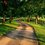# [Geometry] Condition on the Vertex Configurations for an Archimedean Solid

An Archimedean solid has a vertex configuration $\{n_1, n_2, ... , n_k\}$ if each vertex borders regular polygons with $n_i$ number of sides, $i=1,..., k$. For example, $\{3,5,3,5\}$ will give a vertex belonging to four distinct faces, where each will be alternating triangles and pentagons.

Let's consider an Archimedean solid have a vertex configuration $\{ n_1, ... , n_k\}$. We consider the internal angle of a regular polygon, which is given by $\pi - \frac{2\pi}{n}$. This means that at any vertex, the sum of internal angles of the $n_i$-gons can be expressed as $\sum_{i=1}^{k} \left( 1-\frac{2}{n_i}\right) < 2.$ since the sum of internal face angles cannot exceed $2\pi$ at each vertex. If we divide through by $\pi$ on both sides of the equation, we have the following formula,

$\sum_{i=1}{k}\left( 1-\frac{2}{n_i} \right) <2.$

The smallest regular polygon we can get is a triangle, with internal angle 60 degrees, $\frac{2\pi}{6}$ radians at each corner. If we suppose that $k=6$, the sum of internal angles of the $n_i$-gons therefore, is a minimum, of $\frac{2\pi}{6}\cdot 6 = 2\pi$, but the sum of face angles at a vertex must not exceed $2\pi$ or be equal to $2\pi$ since this would create a flat plane and we cannot construct a solid. Hence k cannot be greater than or equal to 6, meaning $k \leq 5.$

Additionally, we see that $k\geq 3$ because if there are any two polygons glued together at any vertex or side, the solid will fall flat and fold into itself.

Therefore, $3\leq k \leq 5$.Note by Bright Glow
3 years, 1 month ago

This discussion board is a place to discuss our Daily Challenges and the math and science related to those challenges. Explanations are more than just a solution — they should explain the steps and thinking strategies that you used to obtain the solution. Comments should further the discussion of math and science.

When posting on Brilliant:

• Use the emojis to react to an explanation, whether you're congratulating a job well done , or just really confused .
• Ask specific questions about the challenge or the steps in somebody's explanation. Well-posed questions can add a lot to the discussion, but posting "I don't understand!" doesn't help anyone.
• Try to contribute something new to the discussion, whether it is an extension, generalization or other idea related to the challenge.
• Stay on topic — we're all here to learn more about math and science, not to hear about your favorite get-rich-quick scheme or current world events.

MarkdownAppears as
*italics* or _italics_ italics
**bold** or __bold__ bold
- bulleted- list
• bulleted
• list
1. numbered2. list
1. numbered
2. list
Note: you must add a full line of space before and after lists for them to show up correctly
paragraph 1paragraph 2

paragraph 1

paragraph 2

[example link](https://brilliant.org)example link
> This is a quote
This is a quote
    # I indented these lines
# 4 spaces, and now they show
# up as a code block.

print "hello world"
# I indented these lines
# 4 spaces, and now they show
# up as a code block.

print "hello world"
MathAppears as
Remember to wrap math in $$ ... $$ or $ ... $ to ensure proper formatting.
2 \times 3 $2 \times 3$
2^{34} $2^{34}$
a_{i-1} $a_{i-1}$
\frac{2}{3} $\frac{2}{3}$
\sqrt{2} $\sqrt{2}$
\sum_{i=1}^3 $\sum_{i=1}^3$
\sin \theta $\sin \theta$
\boxed{123} $\boxed{123}$

## Comments

There are no comments in this discussion.

×

Problem Loading...

Note Loading...

Set Loading...